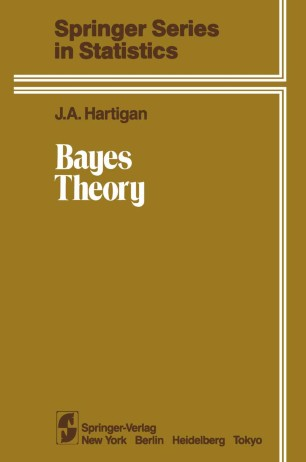# Bayes Theory

• J. A. HartiganBook

Part of the Springer Series in Statistics book series (SSS)

1. Front Matter
Pages i-xii
2. J. A. Hartigan
Pages 1-13
3. J. A. Hartigan
Pages 14-22
4. J. A. Hartigan
Pages 23-33
5. J. A. Hartigan
Pages 34-43
6. J. A. Hartigan
Pages 44-55
7. J. A. Hartigan
Pages 56-62
8. J. A. Hartigan
Pages 63-71
9. J. A. Hartigan
Pages 72-83
10. J. A. Hartigan
Pages 84-95
11. J. A. Hartigan
Pages 96-106
12. J. A. Hartigan
Pages 107-118
13. J. A. Hartigan
Pages 119-126
14. J. A. Hartigan
Pages 127-135
15. Back Matter
Pages 137-145

### Introduction

This book is based on lectures given at Yale in 1971-1981 to students prepared with a course in measure-theoretic probability. It contains one technical innovation-probability distributions in which the total probability is infinite. Such improper distributions arise embarras­ singly frequently in Bayes theory, especially in establishing correspondences between Bayesian and Fisherian techniques. Infinite probabilities create interesting complications in defining conditional probability and limit concepts. The main results are theoretical, probabilistic conclusions derived from probabilistic assumptions. A useful theory requires rules for constructing and interpreting probabilities. Probabilities are computed from similarities, using a formalization of the idea that the future will probably be like the past. Probabilities are objectively derived from similarities, but similarities are sUbjective judgments of individuals. Of course the theorems remain true in any interpretation of probability that satisfies the formal axioms. My colleague David Potlard helped a lot, especially with Chapter 13. Dan Barry read proof. vii Contents CHAPTER 1 Theories of Probability 1. 0. Introduction 1 1. 1. Logical Theories: Laplace 1 1. 2. Logical Theories: Keynes and Jeffreys 2 1. 3. Empirical Theories: Von Mises 3 1. 4. Empirical Theories: Kolmogorov 5 1. 5. Empirical Theories: Falsifiable Models 5 1. 6. Subjective Theories: De Finetti 6 7 1. 7. Subjective Theories: Good 8 1. 8. All the Probabilities 10 1. 9. Infinite Axioms 11 1. 10. Probability and Similarity 1. 11. References 13 CHAPTER 2 Axioms 14 2. 0. Notation 14 2. 1. Probability Axioms 14 2. 2.

### Keywords

Bayessches Verfahren Finite Logic boundary element method conditional probability form innovation object probability probability axioms probability distribution proof similarity techniques theorem

#### Authors and affiliations

• J. A. Hartigan
• 1
1. 1.Department of StatisticsYale UniversityNew HavenUSA

### Bibliographic information

• DOI https://doi.org/10.1007/978-1-4613-8242-3
• Copyright Information Springer-Verlag New York 1983
• Publisher Name Springer, New York, NY
• eBook Packages
• Print ISBN 978-1-4613-8244-7
• Online ISBN 978-1-4613-8242-3
• Series Print ISSN 0172-7397
• Buy this book on publisher's site